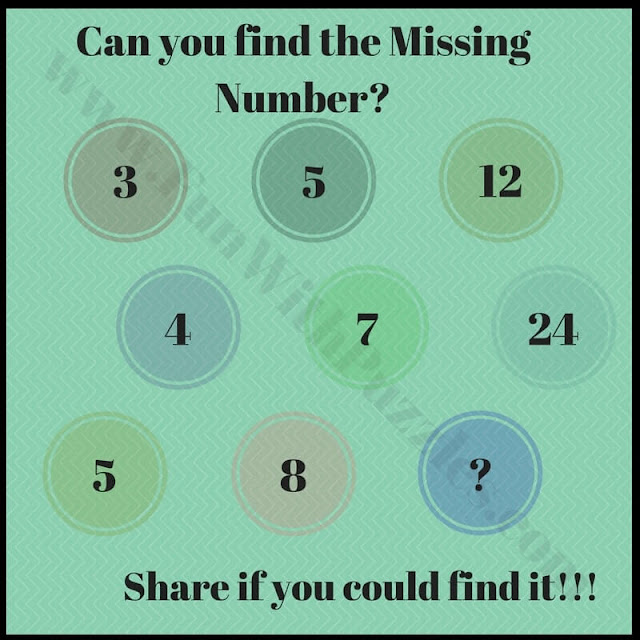Mathematical Puzzles are very fun to solve especially when these Maths Puzzles are represented in pictures. Kids love to solve these Mathematical Puzzles. Here I am posting some of the Mathematical Puzzles which are easy so that kids can solve these puzzles rapidly.
In these Rapidly Easy Maths Puzzles, some numbers are given in/or a Mathematical Shape. These numbers are related to each other with some easy Mathematical pattern. You have to find this Maths pattern and then find the missing number which will replace the question mark.
Answers to these Rapid Maths Puzzles are given at the end. Also, links to similar Maths Puzzles are given after each Puzzle Picture.1. Can you find the missing number?

## Math brain teasers with answers for high school2. Can you find the missing number in this puzzle?

## Simple maths puzzles with answers for kids3. Which number will replace the question mark?

## Find the Missing Numbers Maths Brain Teasers4. Which number will come at the place of the question mark?

## Mathematical brain teasers5. Can you find the missing numbers?

Please check out below mentioned Math Brain Teasers to challenge your brain

## Clever Maths Brain Teasers with answers

Check out some of the clever Maths Brain Teasers which will tickle your mind.

## Mathematical Logical Puzzles for Kids and Teens

These are Mathematical Reasoning Puzzles in which you will not only require your Mathematical skills but also Logical Reasoning.

## Basic Twist Practical problems with answers

Try to solve some practical problems. Give your best before you look at the answers before these problems do have remarkable solutions.